Anzeige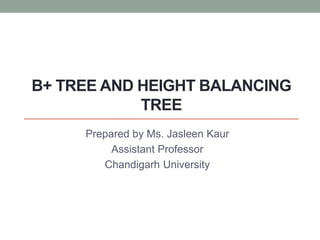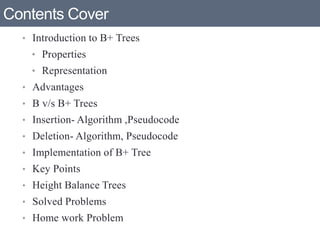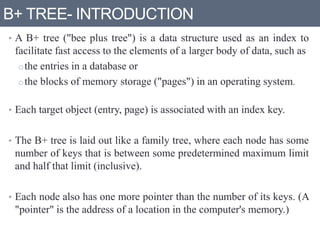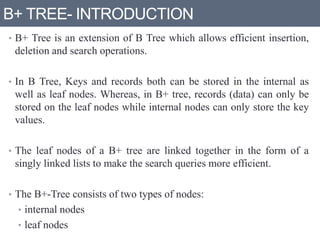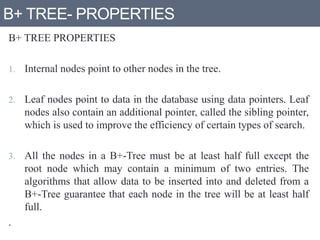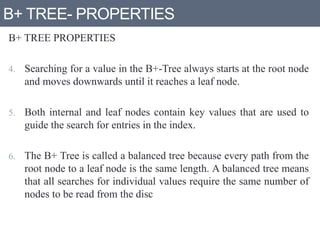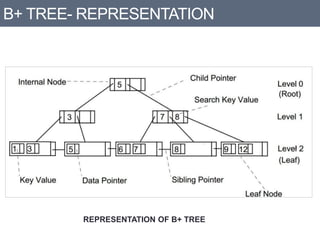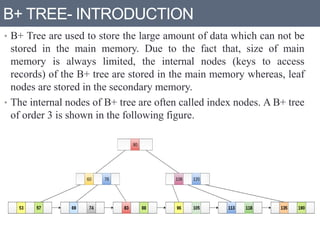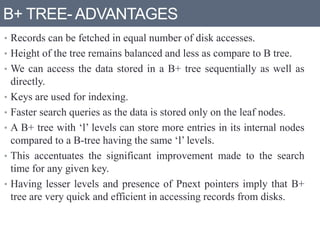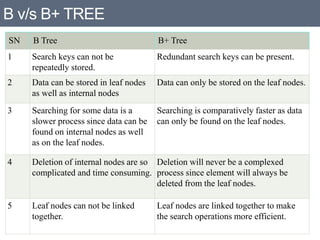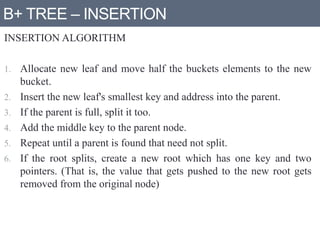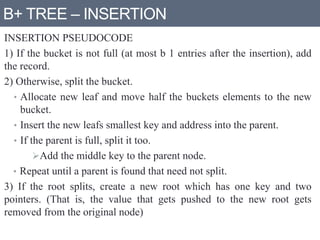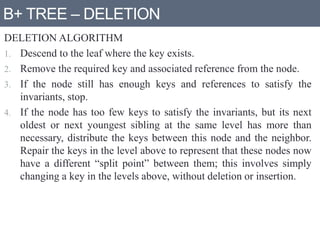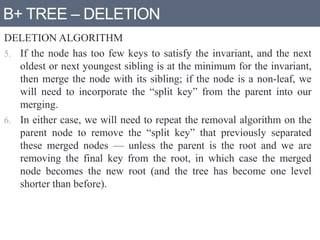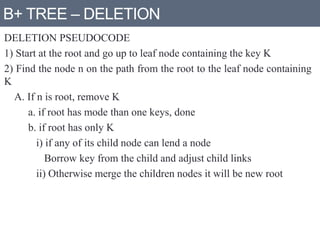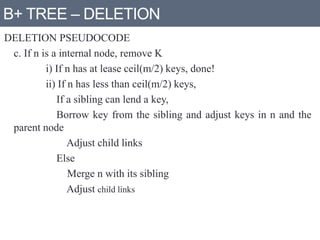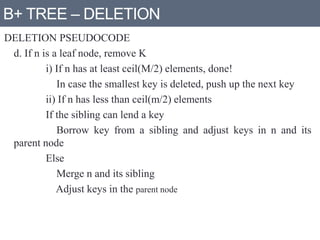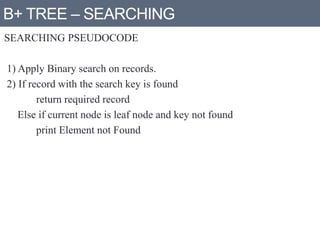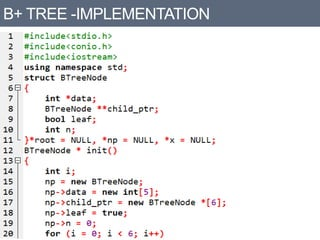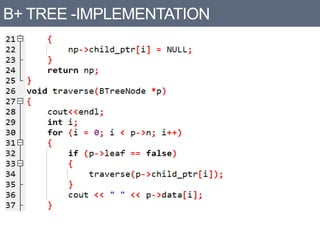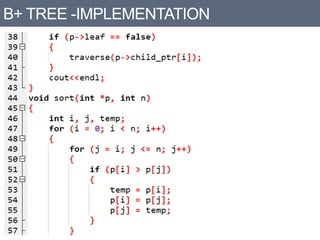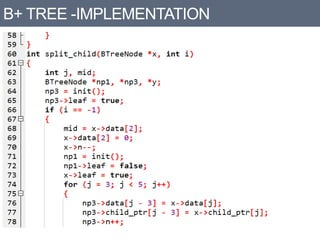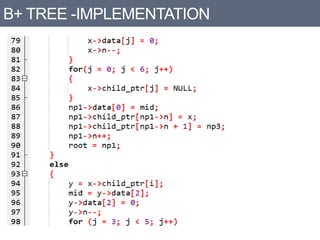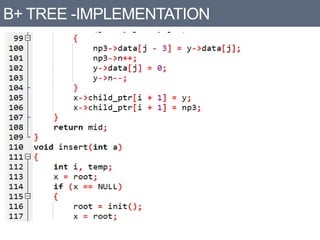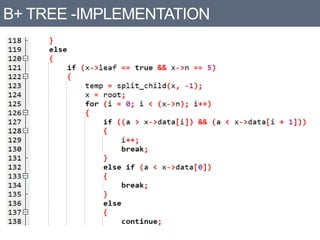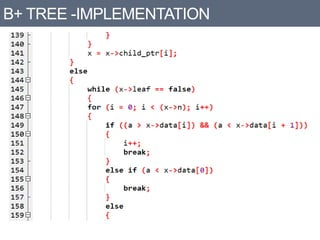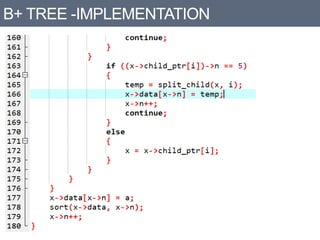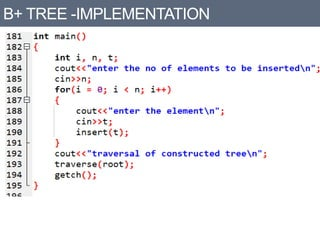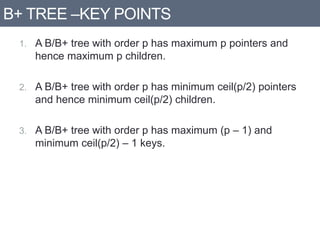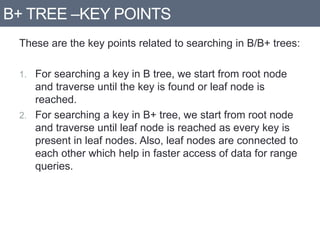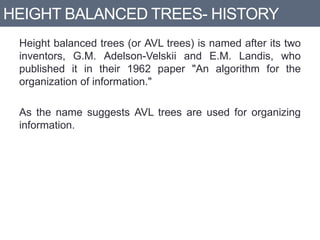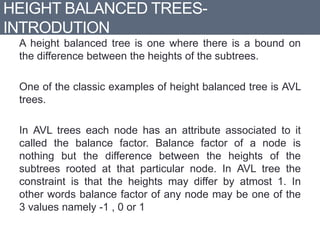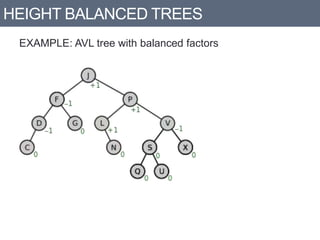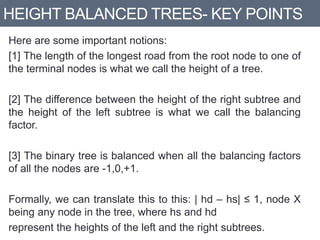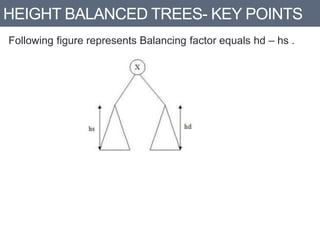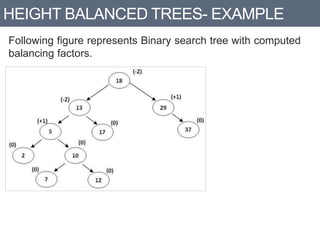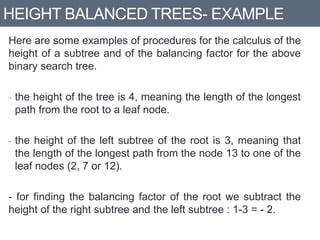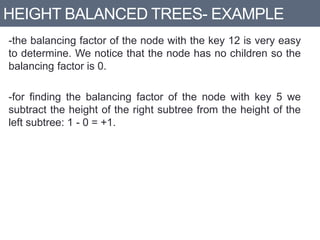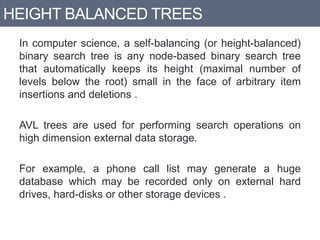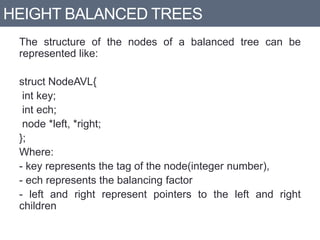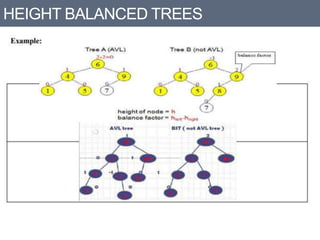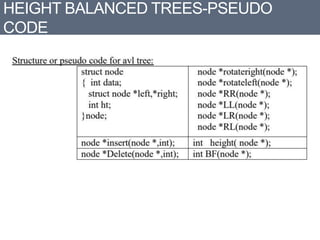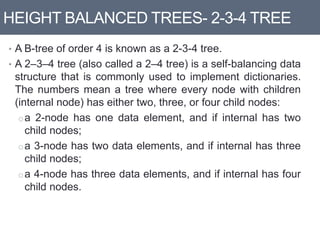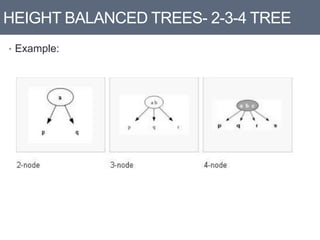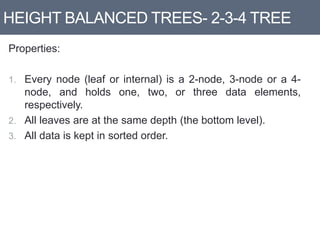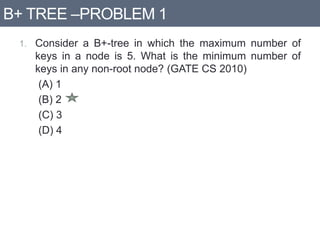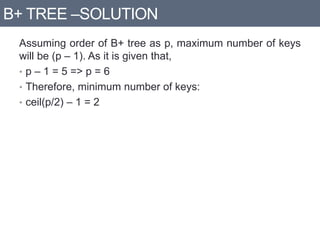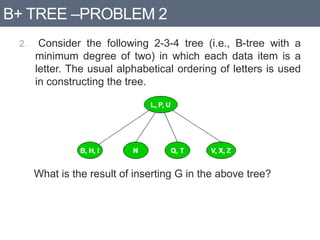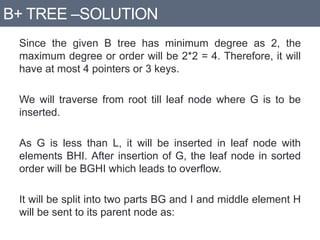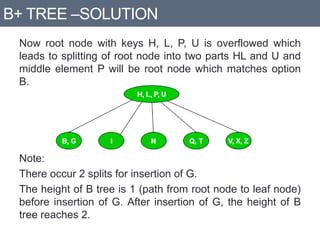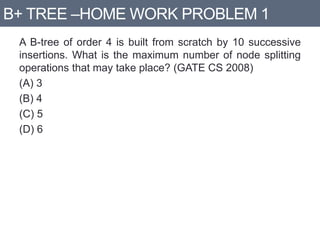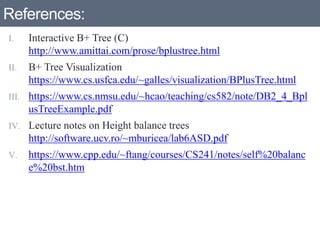1 von 52
Anzeige

### B+ trees and height balance tree

1. B+ TREE AND HEIGHT BALANCING TREE Prepared by Ms. Jasleen Kaur Assistant Professor Chandigarh University
2. Contents Cover • Introduction to B+ Trees • Properties • Representation • Advantages • B v/s B+ Trees • Insertion- Algorithm ,Pseudocode • Deletion- Algorithm, Pseudocode • Implementation of B+ Tree • Key Points • Height Balance Trees • Solved Problems • Home work Problem
3. B+ TREE- INTRODUCTION • A B+ tree ("bee plus tree") is a data structure used as an index to facilitate fast access to the elements of a larger body of data, such as othe entries in a database or othe blocks of memory storage ("pages") in an operating system. • Each target object (entry, page) is associated with an index key. • The B+ tree is laid out like a family tree, where each node has some number of keys that is between some predetermined maximum limit and half that limit (inclusive). • Each node also has one more pointer than the number of its keys. (A "pointer" is the address of a location in the computer's memory.)
4. B+ TREE- INTRODUCTION • B+ Tree is an extension of B Tree which allows efficient insertion, deletion and search operations. • In B Tree, Keys and records both can be stored in the internal as well as leaf nodes. Whereas, in B+ tree, records (data) can only be stored on the leaf nodes while internal nodes can only store the key values. • The leaf nodes of a B+ tree are linked together in the form of a singly linked lists to make the search queries more efficient. • The B+-Tree consists of two types of nodes: • internal nodes • leaf nodes
5. B+ TREE- PROPERTIES B+ TREE PROPERTIES 1. Internal nodes point to other nodes in the tree. 2. Leaf nodes point to data in the database using data pointers. Leaf nodes also contain an additional pointer, called the sibling pointer, which is used to improve the efficiency of certain types of search. 3. All the nodes in a B+-Tree must be at least half full except the root node which may contain a minimum of two entries. The algorithms that allow data to be inserted into and deleted from a B+-Tree guarantee that each node in the tree will be at least half full. .
6. B+ TREE- PROPERTIES B+ TREE PROPERTIES 4. Searching for a value in the B+-Tree always starts at the root node and moves downwards until it reaches a leaf node. 5. Both internal and leaf nodes contain key values that are used to guide the search for entries in the index. 6. The B+ Tree is called a balanced tree because every path from the root node to a leaf node is the same length. A balanced tree means that all searches for individual values require the same number of nodes to be read from the disc
7. B+ TREE- REPRESENTATION REPRESENTATION OF B+ TREE
8. B+ TREE- INTRODUCTION • B+ Tree are used to store the large amount of data which can not be stored in the main memory. Due to the fact that, size of main memory is always limited, the internal nodes (keys to access records) of the B+ tree are stored in the main memory whereas, leaf nodes are stored in the secondary memory. • The internal nodes of B+ tree are often called index nodes. A B+ tree of order 3 is shown in the following figure.
9. B+ TREE- ADVANTAGES • Records can be fetched in equal number of disk accesses. • Height of the tree remains balanced and less as compare to B tree. • We can access the data stored in a B+ tree sequentially as well as directly. • Keys are used for indexing. • Faster search queries as the data is stored only on the leaf nodes. • A B+ tree with ‘l’ levels can store more entries in its internal nodes compared to a B-tree having the same ‘l’ levels. • This accentuates the significant improvement made to the search time for any given key. • Having lesser levels and presence of Pnext pointers imply that B+ tree are very quick and efficient in accessing records from disks.
10. B v/s B+ TREE SN B Tree B+ Tree 1 Search keys can not be repeatedly stored. Redundant search keys can be present. 2 Data can be stored in leaf nodes as well as internal nodes Data can only be stored on the leaf nodes. 3 Searching for some data is a slower process since data can be found on internal nodes as well as on the leaf nodes. Searching is comparatively faster as data can only be found on the leaf nodes. 4 Deletion of internal nodes are so complicated and time consuming. Deletion will never be a complexed process since element will always be deleted from the leaf nodes. 5 Leaf nodes can not be linked together. Leaf nodes are linked together to make the search operations more efficient.
11. B+ TREE – INSERTION INSERTION ALGORITHM 1. Allocate new leaf and move half the buckets elements to the new bucket. 2. Insert the new leaf's smallest key and address into the parent. 3. If the parent is full, split it too. 4. Add the middle key to the parent node. 5. Repeat until a parent is found that need not split. 6. If the root splits, create a new root which has one key and two pointers. (That is, the value that gets pushed to the new root gets removed from the original node)
12. B+ TREE – INSERTION INSERTION PSEUDOCODE 1) If the bucket is not full (at most b 1 entries after the insertion), add the record. 2) Otherwise, split the bucket. • Allocate new leaf and move half the buckets elements to the new bucket. • Insert the new leafs smallest key and address into the parent. • If the parent is full, split it too. Add the middle key to the parent node. • Repeat until a parent is found that need not split. 3) If the root splits, create a new root which has one key and two pointers. (That is, the value that gets pushed to the new root gets removed from the original node)
13. B+ TREE – DELETION DELETION ALGORITHM 1. Descend to the leaf where the key exists. 2. Remove the required key and associated reference from the node. 3. If the node still has enough keys and references to satisfy the invariants, stop. 4. If the node has too few keys to satisfy the invariants, but its next oldest or next youngest sibling at the same level has more than necessary, distribute the keys between this node and the neighbor. Repair the keys in the level above to represent that these nodes now have a different “split point” between them; this involves simply changing a key in the levels above, without deletion or insertion.
14. B+ TREE – DELETION DELETION ALGORITHM 5. If the node has too few keys to satisfy the invariant, and the next oldest or next youngest sibling is at the minimum for the invariant, then merge the node with its sibling; if the node is a non-leaf, we will need to incorporate the “split key” from the parent into our merging. 6. In either case, we will need to repeat the removal algorithm on the parent node to remove the “split key” that previously separated these merged nodes — unless the parent is the root and we are removing the final key from the root, in which case the merged node becomes the new root (and the tree has become one level shorter than before).
15. B+ TREE – DELETION DELETION PSEUDOCODE 1) Start at the root and go up to leaf node containing the key K 2) Find the node n on the path from the root to the leaf node containing K A. If n is root, remove K a. if root has mode than one keys, done b. if root has only K i) if any of its child node can lend a node Borrow key from the child and adjust child links ii) Otherwise merge the children nodes it will be new root
16. B+ TREE – DELETION DELETION PSEUDOCODE c. If n is a internal node, remove K i) If n has at lease ceil(m/2) keys, done! ii) If n has less than ceil(m/2) keys, If a sibling can lend a key, Borrow key from the sibling and adjust keys in n and the parent node Adjust child links Else Merge n with its sibling Adjust child links
17. B+ TREE – DELETION DELETION PSEUDOCODE d. If n is a leaf node, remove K i) If n has at least ceil(M/2) elements, done! In case the smallest key is deleted, push up the next key ii) If n has less than ceil(m/2) elements If the sibling can lend a key Borrow key from a sibling and adjust keys in n and its parent node Else Merge n and its sibling Adjust keys in the parent node
18. B+ TREE – SEARCHING SEARCHING PSEUDOCODE 1) Apply Binary search on records. 2) If record with the search key is found return required record Else if current node is leaf node and key not found print Element not Found
19. B+ TREE -IMPLEMENTATION
20. B+ TREE -IMPLEMENTATION
21. B+ TREE -IMPLEMENTATION
22. B+ TREE -IMPLEMENTATION
23. B+ TREE -IMPLEMENTATION
24. B+ TREE -IMPLEMENTATION
25. B+ TREE -IMPLEMENTATION
26. B+ TREE -IMPLEMENTATION
27. B+ TREE -IMPLEMENTATION
28. B+ TREE -IMPLEMENTATION
29. B+ TREE –KEY POINTS 1. A B/B+ tree with order p has maximum p pointers and hence maximum p children. 2. A B/B+ tree with order p has minimum ceil(p/2) pointers and hence minimum ceil(p/2) children. 3. A B/B+ tree with order p has maximum (p – 1) and minimum ceil(p/2) – 1 keys.
30. B+ TREE –KEY POINTS These are the key points related to searching in B/B+ trees: 1. For searching a key in B tree, we start from root node and traverse until the key is found or leaf node is reached. 2. For searching a key in B+ tree, we start from root node and traverse until leaf node is reached as every key is present in leaf nodes. Also, leaf nodes are connected to each other which help in faster access of data for range queries.
31. HEIGHT BALANCED TREES- HISTORY Height balanced trees (or AVL trees) is named after its two inventors, G.M. Adelson-Velskii and E.M. Landis, who published it in their 1962 paper "An algorithm for the organization of information." As the name suggests AVL trees are used for organizing information.
32. HEIGHT BALANCED TREES- INTRODUTION A height balanced tree is one where there is a bound on the difference between the heights of the subtrees. One of the classic examples of height balanced tree is AVL trees. In AVL trees each node has an attribute associated to it called the balance factor. Balance factor of a node is nothing but the difference between the heights of the subtrees rooted at that particular node. In AVL tree the constraint is that the heights may differ by atmost 1. In other words balance factor of any node may be one of the 3 values namely -1 , 0 or 1
33. HEIGHT BALANCED TREES EXAMPLE: AVL tree with balanced factors
34. HEIGHT BALANCED TREES- KEY POINTS Here are some important notions:  The length of the longest road from the root node to one of the terminal nodes is what we call the height of a tree.  The difference between the height of the right subtree and the height of the left subtree is what we call the balancing factor.  The binary tree is balanced when all the balancing factors of all the nodes are -1,0,+1. Formally, we can translate this to this: | hd – hs| ≤ 1, node X being any node in the tree, where hs and hd represent the heights of the left and the right subtrees.
35. HEIGHT BALANCED TREES- KEY POINTS Following figure represents Balancing factor equals hd – hs .
36. HEIGHT BALANCED TREES- EXAMPLE Following figure represents Binary search tree with computed balancing factors.
37. HEIGHT BALANCED TREES- EXAMPLE Here are some examples of procedures for the calculus of the height of a subtree and of the balancing factor for the above binary search tree. - the height of the tree is 4, meaning the length of the longest path from the root to a leaf node. - the height of the left subtree of the root is 3, meaning that the length of the longest path from the node 13 to one of the leaf nodes (2, 7 or 12). - for finding the balancing factor of the root we subtract the height of the right subtree and the left subtree : 1-3 = - 2.
38. HEIGHT BALANCED TREES- EXAMPLE -the balancing factor of the node with the key 12 is very easy to determine. We notice that the node has no children so the balancing factor is 0. -for finding the balancing factor of the node with key 5 we subtract the height of the right subtree from the height of the left subtree: 1 - 0 = +1.
39. HEIGHT BALANCED TREES In computer science, a self-balancing (or height-balanced) binary search tree is any node-based binary search tree that automatically keeps its height (maximal number of levels below the root) small in the face of arbitrary item insertions and deletions . AVL trees are used for performing search operations on high dimension external data storage. For example, a phone call list may generate a huge database which may be recorded only on external hard drives, hard-disks or other storage devices .
40. HEIGHT BALANCED TREES The structure of the nodes of a balanced tree can be represented like: struct NodeAVL{ int key; int ech; node *left, *right; }; Where: - key represents the tag of the node(integer number), - ech represents the balancing factor - left and right represent pointers to the left and right children
41. HEIGHT BALANCED TREES
42. HEIGHT BALANCED TREES-PSEUDO CODE
43. HEIGHT BALANCED TREES- 2-3-4 TREE • A B-tree of order 4 is known as a 2-3-4 tree. • A 2–3–4 tree (also called a 2–4 tree) is a self-balancing data structure that is commonly used to implement dictionaries. The numbers mean a tree where every node with children (internal node) has either two, three, or four child nodes: oa 2-node has one data element, and if internal has two child nodes; oa 3-node has two data elements, and if internal has three child nodes; oa 4-node has three data elements, and if internal has four child nodes.
44. HEIGHT BALANCED TREES- 2-3-4 TREE • Example:
45. HEIGHT BALANCED TREES- 2-3-4 TREE Properties: 1. Every node (leaf or internal) is a 2-node, 3-node or a 4- node, and holds one, two, or three data elements, respectively. 2. All leaves are at the same depth (the bottom level). 3. All data is kept in sorted order.
46. B+ TREE –PROBLEM 1 1. Consider a B+-tree in which the maximum number of keys in a node is 5. What is the minimum number of keys in any non-root node? (GATE CS 2010) (A) 1 (B) 2 (C) 3 (D) 4
47. B+ TREE –SOLUTION Assuming order of B+ tree as p, maximum number of keys will be (p – 1). As it is given that, • p – 1 = 5 => p = 6 • Therefore, minimum number of keys: • ceil(p/2) – 1 = 2
48. B+ TREE –PROBLEM 2 2. Consider the following 2-3-4 tree (i.e., B-tree with a minimum degree of two) in which each data item is a letter. The usual alphabetical ordering of letters is used in constructing the tree. What is the result of inserting G in the above tree?
49. B+ TREE –SOLUTION Since the given B tree has minimum degree as 2, the maximum degree or order will be 2*2 = 4. Therefore, it will have at most 4 pointers or 3 keys. We will traverse from root till leaf node where G is to be inserted. As G is less than L, it will be inserted in leaf node with elements BHI. After insertion of G, the leaf node in sorted order will be BGHI which leads to overflow. It will be split into two parts BG and I and middle element H will be sent to its parent node as:
50. B+ TREE –SOLUTION Now root node with keys H, L, P, U is overflowed which leads to splitting of root node into two parts HL and U and middle element P will be root node which matches option B. Note: There occur 2 splits for insertion of G. The height of B tree is 1 (path from root node to leaf node) before insertion of G. After insertion of G, the height of B tree reaches 2.
51. B+ TREE –HOME WORK PROBLEM 1 A B-tree of order 4 is built from scratch by 10 successive insertions. What is the maximum number of node splitting operations that may take place? (GATE CS 2008) (A) 3 (B) 4 (C) 5 (D) 6
52. References: I. Interactive B+ Tree (C) http://www.amittai.com/prose/bplustree.html II. B+ Tree Visualization https://www.cs.usfca.edu/~galles/visualization/BPlusTree.html III. https://www.cs.nmsu.edu/~hcao/teaching/cs582/note/DB2_4_Bpl usTreeExample.pdf IV. Lecture notes on Height balance trees http://software.ucv.ro/~mburicea/lab6ASD.pdf V. https://www.cpp.edu/~ftang/courses/CS241/notes/self%20balanc e%20bst.htm
Anzeige